﻿ 一种新的谱聚类算法及其仿真 A New Spectral Clustering Algorithm and Its Simulation

Computer Science and Application
Vol.07 No.11(2017), Article ID:22797,11 pages
10.12677/CSA.2017.711125

A New Spectral Clustering Algorithm and Its Simulation

Jiao Li, Xuan Wang*

School of Physics and Information Technology, Shaanxi Normal University, Xi’an Shaanxi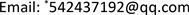Received: Nov. 7th, 2017; accepted: Nov. 20th, 2017; published: Nov. 27th, 2017ABSTRACT

The similarity between the samples based on Euclidean distance measurement can not reflect the probability distribution characteristics of the samples, especially the sample clustering with multi-peak distribution, and the Euclidean distance has a large deviation. In addition, the k-means algorithm widely used in the class often produces unstable clustering results due to the randomness of the initial center. In this paper, a similarity measure method which can reflect the probability distribution of the sample is proposed for the similarity measure. On this basis, the k-means algorithm in spectral clustering is improved, and a method is proposed for the initial center adaptive setting method. The experimental results show that the proposed algorithm has better clustering effect than the traditional spectral clustering in the artificial data set and the UCI real data set.

Keywords:Spectral Graph Theory, Spectral Clustering, Similarity Matrix, k-Means1. 引言

2. 算法回顾

Step 1：构建表示样本集的矩阵Z；

Step 2：通过计算Z的前k个特征值与特征向量，构建特征向量空间；

Step 3：利用k-means聚类算法对特征向量空间中的特征向量进行聚类。

(1) 根据欧氏距离量度构造相似性矩阵 ${S}_{ij}=\mathrm{exp}\left(-\frac{dist}{2{\sigma }^{2}}\right)$

(2) 构造拉普拉斯矩阵 $L={D}^{-\frac{1}{2}}S{D}^{-\frac{1}{2}}$ ，其中 $D$ 为对角矩阵；

(3) 求拉普拉斯 $L$$k$ 个最大特征值对应的特征向量 ${v}_{1},{v}_{2},{v}_{3},\cdots ,{v}_{k}$ ，并且构造矩阵 $V=\left[{v}_{1},{v}_{2},\cdots ,{v}_{k}\right]\in {R}^{n×k}$ ，其中 ${v}_{k}$ 为列向量；

(4) 单位化 $V$ 的行向量，得到矩阵 $Y$ ，其中 ${Y}_{ij}=\frac{{V}_{ij}}{{\left(\underset{j}{\sum }{V}_{ij}^{2}\right)}^{\frac{1}{2}}}$

(5) 将 $Y$ 的每一行看成是 ${R}^{k}$ 空间内的一点，使用K均值将其聚为 $k$ 类；

(6) 如果 $Y$ 的第 $i$ 行属于第 $j$ 类，则将原始数据点 ${x}_{i}$ 也划分到第 $j$ 类。

3. 改进的算法

3.1. 优化的相似性度量

$L\left({x}_{i},{x}_{j}\right)={\rho }^{d\left({x}_{i},{x}_{j}\right)}-1$ (1)

${D}_{ij}=\underset{p\in {p}_{j}}{\mathrm{min}}\underset{k=1}{\overset{l-1}{\sum }}L\left({p}_{k},{p}_{k+1}\right)$ (2)

3.2. 优化的K-means算法

3.3. 改进的谱聚类算法

(1) 根据密度敏感的相似性度量构造相似性矩阵 $W\in {R}^{n×n}$

(2) 构造拉普拉斯矩阵 $L={D}^{-\frac{1}{2}}S{D}^{-\frac{1}{2}}$ ，其中 $D$ 为对角矩阵， ${D}_{ii}=\underset{j=1}{\overset{n}{\sum }}{W}_{ij}$

(3) 求拉普拉斯 $L$$k$ 个最大特征值对应的特征向量 ${v}_{1},{v}_{2},{v}_{3},\cdots ,{v}_{k}$ ，并且构造矩阵 $V=\left[{v}_{1},{v}_{2},\cdots ,{v}_{k}\right]\in {R}^{n×k}$ ，其中 ${v}_{k}$ 为列向量；

(4) 单位化 $V$ 的行向量，得到矩阵 $Y$ ，其中 ${Y}_{ij}=\frac{{V}_{ij}}{{\left(\underset{j}{\sum }{V}_{ij}^{2}\right)}^{\frac{1}{2}}}$

(5) 将 $Y$ 的每一行看成是 ${R}^{k}$ 空间内的一点，使用改进的K均值算法将其聚为 $k$ 类；

(6) 如果 $Y$ 的第 $i$ 行属于第 $j$ 类，则将原始数据点 ${x}_{i}$ 也划分到第 $j$ 类。

4. 实验仿真

4.1. 人工数据集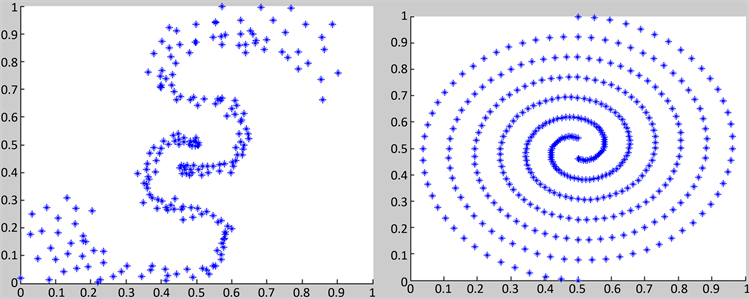(a) (b)(c)

Figure 1. The original datasets

4.2. UCI数据集

UCI数据集是一个用于机器学习的常用标准测试集，是University of California Irvine提出的真实数据集。下面选用该测试集中4个真实数据集进一步验证本文提出算法的优越性，具体数据特征如表1所示。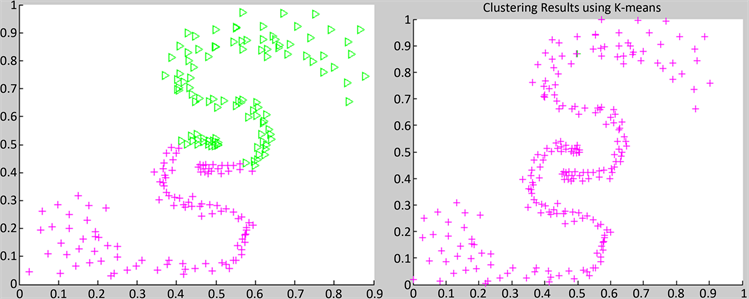(a) (b)(c) (d)

Figure 2. Contrast of four algorithms on synthetic datasets TwoS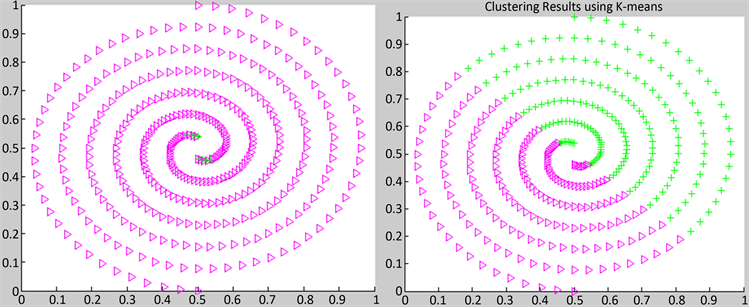(a) (b)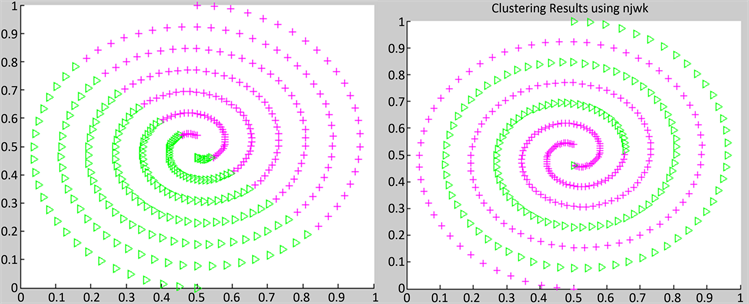(c) (d)

Figure 3. Contrast of four algorithms on synthetic datasets 2Spiral(a) (b)(c) (d)

Figure 4. Contrast of four algorithms on synthetic datasets circle dataTable 1. Experiment dataset and data characteristics

$RI=\frac{TP+TN}{TP+FP+FN+TN}$ (3)

$P\left(i,j\right)=\frac{{n}_{ij}}{{n}_{j}}$ (4)

$R\left(i,j\right)=\frac{{n}_{ij}}{{n}_{i}}$ (5)

$F\left(i,j\right)=\frac{2×P\left(i,j\right)×R\left(i,j\right)}{P\left(i,j\right)+R\left(i,j\right)}$ (6)

$F=\underset{i}{\sum }\frac{{n}_{i}}{n}\underset{j}{\mathrm{max}}\left\{F\left(i,j\right)\right\}$ (7)

$F\in \left[0,1\right]$ , $F$ 值越高表明聚类效果越好。图6表示的是k-means聚类算法、传统聚类算法、密度敏感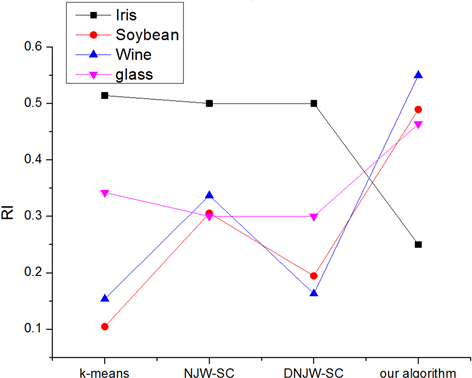Figure 5. Comparison of the RI indicator figures of the four sets of data clustered by the four algorithms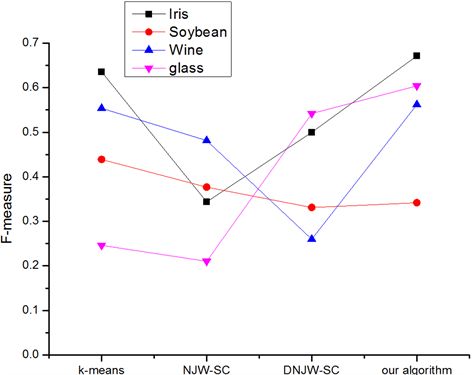Figure 6. Comparison of the F-measure indicator figures of the four sets of data clustered by the four algorithms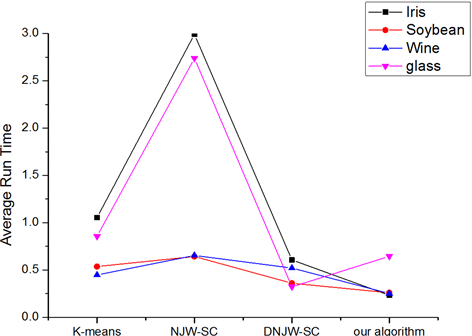Figure 7. Comparison of average run time of the four sets of data clustered by the four algorithms

5. 结论

A New Spectral Clustering Algorithm and Its Simulation[J]. 计算机科学与应用, 2017, 07(11): 1107-1117. http://dx.doi.org/10.12677/CSA.2017.711125

1. 1. Jain, A., Murty, M. and Flynn, P. (1999) Data Clustering: A Review. ACM Computing Surveys, 31, 264-323. https://doi.org/10.1145/331499.331504

2. 2. 王晅, 马建峰. 数字图像分析与模式识别[M]. 北京: 科学出版社, 2011: 228-229.

3. 3. Huang, Z. (1998) Extensions to the k-Means Algorithm for Clustering Large Datasets with Categorical Values. Data Mining and Knowledge Discovery, 283-304.

4. 4. Wu, X. and Zhang, S. (2003) Synthesizing High-Frequency Rules from Different Data Sources. IEEE Transactions on Knowledge and Data Engineering, 15, 353-367.

5. 5. Wu, X., Zhang, C. and Zhang, S. (2005) Database Classification for Multi-Database Mining. Information Systems, 30, 71-88. https://doi.org/10.1016/j.is.2003.10.001

6. 6. Luxburg, U.V. (2007) A Tutorial on Spectral. Statistics and Computing, 17, 395-416. https://doi.org/10.1007/s11222-007-9033-z

7. 7. Yan, D. (2009) Fast Approximate Spectral Clustering. UC Berkeley, 907-915. https://doi.org/10.1145/1557019.1557118

8. 8. 蔡晓妍, 戴冠中, 等. 谱聚类算法综述[J]. 计算机科学, 2008, 35(7): 14-18.

9. 9. Wu, Z. and Leahy, R. (1993) An Optimal Graph Theoretic Approach to Data Clustering: Theory and Its Application to Image Segmentation. IEEE Transactions on PAMI, 15, 1101-1113.

10. 10. Chang, H. and Yeung, D.Y. (2011) Robust Path-Based Spectral Clustering. Applied and Computational Harmonic Analysis, 30, 319-336.

11. 11. Chi, Y. and Song, X.D. (2009) On Evolutionary Spectral Clustering. ACM Transactions on Knowledge Discovery from Data, 3, Article No. 17. https://doi.org/10.1145/1631162.1631165

12. 12. Tulin, I. (2015) A Parameter-Free Similarity Graph for Spectral Clustering. Expert Systems with Applications, 42, 9489-9498. https://doi.org/10.1016/j.eswa.2015.07.074

13. 13. Mario, B. (2015) A Density-Based Similarity Matrix Construction for Spectral Clustering. Neurocomputing, 151, 835-844. https://doi.org/10.1016/j.neucom.2014.10.012

14. 14. Yan, J., Cheng, D.B., Zong, M. and Deng, Z.Y. (2014) Improved Spectral Clustering Algorithm Based on Similarity Measure. In: Luo, X., Yu, J.X. and Li, Z., Eds., Advanced Data Mining and Applications. ADMA 2014. Lecture Notes in Computer Science, Vol. 8933, Springer, Cham, 641-654. https://doi.org/10.1007/978-3-319-14717-8_50

15. 15. Anil, K.J. (2010) Data Clustering: 50 Years beyond K-Means. Pattern Recognition Letters, 31, 651-666. https://doi.org/10.1016/j.patrec.2009.09.011

16. 16. Ng, A.Y., Jordan, M.I. and Weiss, Y. (2002) On Spectral Clustering: Analysis and an Algorithm. In: Advances in Neural Information Processing Systems, MIT Press, Cambridge, MA, 894-856.

17. 17. Yang, P., Zhu, Q.S. and Huang, B. (2011) Spectral Clustering with Density Sensitive Similarity Function. Knowledge-Based Systems, 24, 621-628. https://doi.org/10.1016/j.knosys.2011.01.009

18. 18. Zhang, X., Jiao, L., Liu, F., et al. (2008) Spectral Clustering Ensemble Applied to SAR Image Segmentation. IEEE Transactions on Geosciences and Remote Sensing, 46, 2126-2136. https://doi.org/10.1109/TGRS.2008.918647

19. 19. Cai, X.Y., Dai, G.Z. and Yang, L.B. (2009) A New Self-Adaptive Clustering Algorithm. China Science and Technology Press, Beijing, 329-334.

20. 20. Fiedler, M. (1975) A Property of Eigenvectors of Non-Negative Symmetric Matrices and Its Application to Graph Theory. Czechoslovak Mathematical Journal, 25, 619-633.